## Basic DC Circuit Analysis Interview Questions and Answers:

1. Define Electric current.

Ans. Electric current is defined as the rate of flow of electric charge.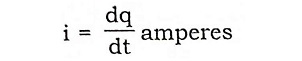Where q is the charge is coulombs. The unit of current is the ampere which is the current that flows when 1 coulomb of charge is transferred in one second.

2. Define Electrical potential or Voltage.

Ans. This is generally measured between two points and its unit is volt. If the work done in moving a charge of one coulomb between any two points is 1 joule, then we say that the potential of one point with reference to the second point is 1 volt.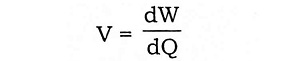where W is the work done in joules.

3. What is meant by resistance?

Ans. The resistance of a circuit is the property by which it opposes the flow of current. This parameter measured in Ohms is responsible for energy dissipation.

4. Define conductance.

Ans. The reciprocal of resistance is called conductance: Its unit is the Siemen and its symbol is G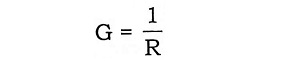5. State Ohm’s law.

Ans. When the temperature remains constant, current flowing through a circuit is directly proportional to potential difference across the conductor.

Mathematically, we may write E ∞ I (Temperature being constant) (or) E = IR.

Where ‘R’ the constant of proportionally, becomes the resistance when E is in Volts and I is in amperes.

6. Define electrical power.

Ans. The rate at which work is done in an electrical circuit is called electrical power and its unit is joule per second or watt. When one coulomb of electric charge is moved through a potential difference of one volt in one second, the rate of work is one joule per second or one watt. Hence power in electric circuits is obtained as a product of the voltage (V) and current (I).

P = V.I Watts

We may write this as

P = I2 R (or)

P = V2/R

7. What is meant by electric energy?

Ans. Electrical energy is the total amount of work done and hence is the product of power and time.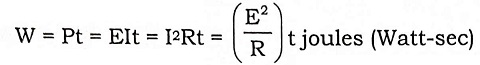8. State Kirchoff’s laws.

Ans.

Kirchoff’s Current Law (I Law)

The sum of the currents flowing towards a junction is equal to the sum of the currents flowing away from it.

Kirchoff’s Voltage Law (II Law)

In a closed circuit, the sum of the potential drops is equal to the sum of the potential rises.

9. Write the general form of mesh analysis.

Ans.

[R] [I] = [V]

10. What are called lumped circuits?

Ans. A network in which all the network elements are physically separable is known as lumped network. Most of the electrical networks are lumped networks.

11. Write the equivalent resistance when two resistors are connected in parallel?

Ans.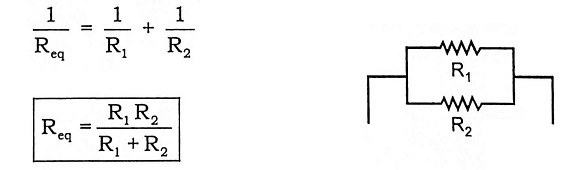12. Write the equivalent resistance when three resistors are connected in series?

Ans.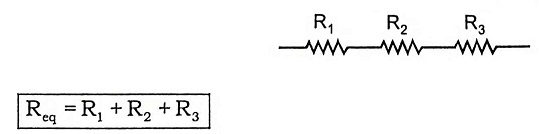13. Mention the limitations of Ohm’s law.

Ans.

1. Ohm’s law does not apply to all non-metallic conductors.
2. It does not also apply to non-linear devices such as zener diode, vacuum tubes etc.
3. Ohm’s law is true for metal conductors at constant temperature. If the temperature changes, the law is not applicable.

14. Give different forms of expression for electrical power.

Ans.

Power P = VI

= I2R

= V2/R

where,

V = voltage, I = current, R = resistance

15. Define linear and bilateral network.

Ans.

Linear Network: A circuit or network whose parameters i.e., elements like resistors, inductors and capacitors are always constant irrespective of the change in time, voltage, temperature etc., is known as linear network. In linear network, the Ohm’s law can be applied and super position theorem can be used for solving mathematical equations.

Bilateral Network: A circuit whose characteristics, behavior is same irrespective of the direction of current through various elements of it, is called bilateral network. Network consisting only resistors is a good example for bilateral network.

16. Define distributed elements. Given an example.

Ans. A network in which the circuit elements like resistor, inductor, etc., cannot be physically separated for analysis purpose, is called distributed network.

For example, in a transmission line where resistance, inductance and capacitance are distributed all along its length. They cannot be shown as separate elements, anywhere in the circuit.

17. Define Non-linear network.

Ans. A circuit where parameters change their values with change in time, temperature, voltage etc., is known as non linear network. Ohm’s law and law of superposition do not apply to this network.

18. Define Unilateral network.

Ans. A circuit whose operation, behavior is dependent on the direction of the current through various elements is called unilateral network. Example for unilateral network is half wave diode rectifier.

19. Define active and passive networks.

Ans.

Active Network: A circuit which contains a source of energy is called active network. Voltage and current sources are energy sources.

Passive Network: A circuit which contains no energy source is called passive circuit. The passive network contains resistor, capacitor and inductor.

20. What are the types of circuit elements?

Ans.

Active element: Voltage and current sources

Passive element: Resistor, inductor, capacitor

21. What is an ideal voltage source and an ideal current source?

Ans.

Ideal Voltage Source : Voltage source is a source which delivers energy with specified terminal voltage, which is independent of the current supplied by the source. Such a voltage source which maintains constant specified voltage for all currents supplied by the source, the internal impedance is zero.

Ideal Current Source : The current source is a source which delivers energy with a specified current which is independent of the voltage at its terminals. Such a current source which maintains a constant specified current for all voltages is called an ideal current source.

22. Draw the V-I relationship of an ideal voltage source.

Ans.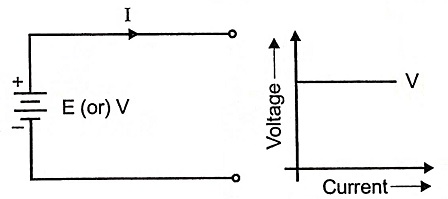23. What are the ideal sources of Electrical energy?

Ans.

1. Ideal voltage source   2. Ideal current source

24. Draw the V-I relationship of an ideal current source.

Ans.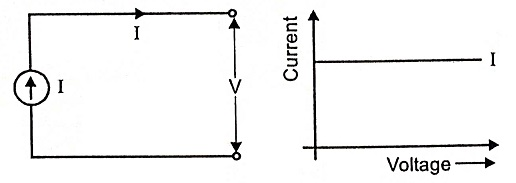25. Draw the V-I relationship of a practical voltage source.

Ans.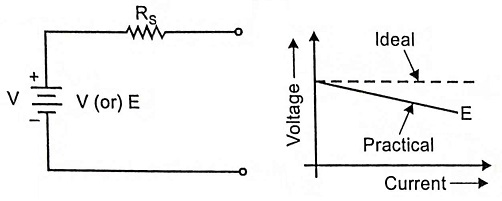26. Draw the V-I relationship of practical current source.

Ans.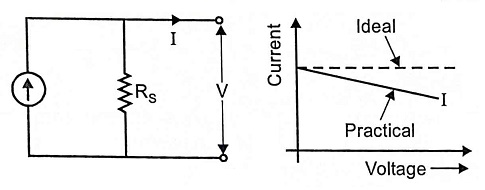27. Write the current division rule.

Ans.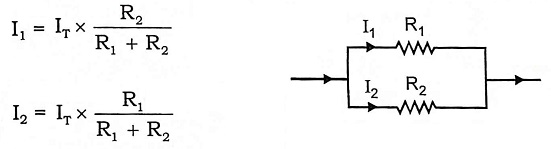28. What is the equivalent resistance when ‘N’ resistors are in (a) series (b) in parallel?

Ans.

(a) Series Connection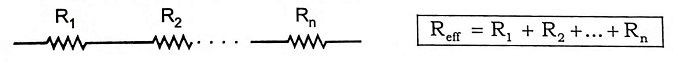(b) Parallel Connection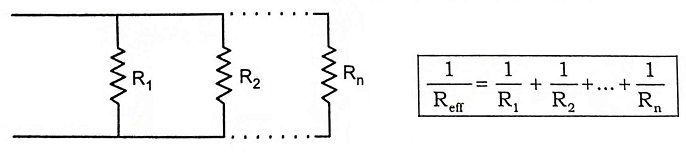29. What do you understand by Nodal analysis?

Ans. The nodal method is used to analyze multisource circuits. This method requires that we solve the simultaneous equations that result when Kirchoff’s current law (KCL) is applied at various nodes in an electric circuit. Node is defined as junction or joining point of two or more component terminals.

Scroll to Top